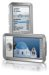# Example 1 - flags and bit insert/delete

Analyzing directly from the bits on the line is not something you will need to do in practice because all protocol testers do this automatically.

However try this example to get an insight into how the datastream is divided into frames.

Here is another example worked through

______________________________________

0100110111111011000000111110100110110100011011101111110110

----><------> ^ <------><---

end of this a zero flag start

last pattern following of next

frame must be five ones frame

a flag must have

wherever n inserted

it occurs for transparency

so remove it

this leaves the following,

1100000011111 1001101101000110111

divide up into bytes,

11000000 11111100 11011010 00110111

<---------------->

the sixteen bits

before the flag is

always the frame

check sequence

<------>

this is the

control byte

the LSB is

transmitted

first so it

is 3F in hex

which identifies

this as a SABM

frame.

<------>

this is

03 in hex

which is

Now try the example yourself - don't worry about what type of frame it is - just decode it to HEX

1= 0001

2= 0010

3= 0011

4= 0100

5= 0101

6= 0110

7= 0111

8= 1000

9= 1001

A= 1010

B= 1011

C= 1100

D= 1101

E= 1110

F= 1111

__________________

1100111111000000011111011110011111100111

<------> ^ <------>

flag bit flag

inserted

flags and

'bit stuffing' 0000001111111110

removed

value in hex C0 7F

(low order bit of

each byte

transmitted first)

all frames must have at least 4 bytes so this is not a valid frame

______________

| slide EX 2 |

______________

* This slide was made up from a printout from a halcyon

(the halcyon screen is the same except it does not have

the line with all thNNNNN's)

* each byte is represented by two hex digits above & below each other e.g.,

4

C = 01001100

* the display is read left to right / top to bottom

* both directions of transmission are displayed

top is from host to network

bottom is from network to host

the trace then continues in pairs like this down the screen

* the flags (7E) are suppressed

apart from the last flag in each frame

* the 16 byte FCS at the end of each frame is suppressed

* - when the draughsman drew out this slide he squashed up some of

the rows so that they do not line up (all frames end with 7E).

__________________

First frame - 03 EA 14 00 02 48 45 4C 4C 4F

- address = 03 = A - network to host so this is a command

- control = EA = 11101010

<->^<->^

| | | +-- this is an I frame

| | +---- N(S)=5

| +------ P=0

+-------- N(R)=7

- packet level is 14 00 02 48 45 4C 4C 4F

- FCS not displayed

Second frame - 01 CE 14 00 41

Address = 01 = B - host to network so this is a command

Control = CE = 11001110

<->^<->^

| | | +-- 0 so this is information frame

| | +---- N(S) = 7

| +------ P=0

+-------- N(R) = 6

Data = 14 00 41

FCS not displayed

Third frame - 01 01

- address = 01 = B - network to host so this is a response

- control = 01 = 00000001

<->^<-->

| | +--- this is an RR frame

| +------ P=0

+-------- N(R)=0

- RR frames do not have an I field

- FCS not displayed

 metadata block see also: Correspondence about this page Book Shop - Further reading. Where I can, I have put links to Amazon for books that are relevant to the subject, click on the appropriate country flag to get more details of the book or to buy it from them. Commercial Software Shop Where I can, I have put links to Amazon for commercial software, not directly related to the software project, but related to the subject being discussed, click on the appropriate country flag to get more details of the software or to buy it from them.Palm LifeDrive - 4GB PDA.

This site may have errors. Don't use for critical systems.